# SSAT Upper Level Math : How to divide fractions

## Example Questions

← Previous 1

### Example Question #1 : How To Divide Fractions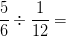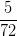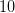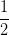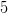Explanation:

Turn the second fraction upside down to find its reciprocal, and then multiply the first fraction by the reciprocal of the second fraction to divide the two fractions. When multiplying fractions, you can multiply across, finding the products of the numbers being multiplied in the numerator and in the denominator.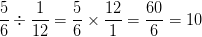### Example Question #2 : How To Divide Fractions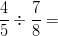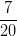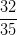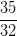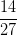Explanation:

Turn the second fraction upside down to find its reciprocal, and then multiply the first fraction by the reciprocal of the second fraction to divide the two fractions. When multiplying fractions, you can multiply across, finding the products of the numbers being multiplied in the numerator and in the denominator.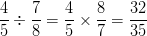### Example Question #3 : How To Divide Fractions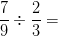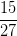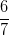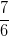Explanation:

Turn the second fraction upside down to find its reciprocal, and then multiply the first fraction by the reciprocal of the second fraction to divide the two fractions. When multiplying fractions, you can multiply across, finding the products of the numbers being multiplied in the numerator and in the denominator.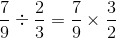In this case, you can reduce the fractions being multiplied by cross-canceling before multiplying them together.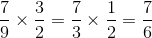### Example Question #4 : How To Divide Fractions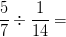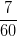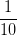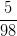Explanation:

Turn the second fraction upside down to find its reciprocal, and then multiply the first fraction by the reciprocal of the second fraction to divide the two fractions. When multiplying fractions, you can multiply across, finding the products of the numbers being multiplied in the numerator and in the denominator.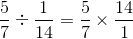In this case, you can reduce the fractions being multiplied by cross-canceling before multiplying them together.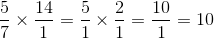### Example Question #5 : How To Divide Fractions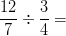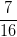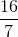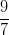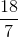Explanation:

Turn the second fraction upside down to find its reciprocal, and then multiply the first fraction by the reciprocal of the second fraction to divide the two fractions. When multiplying fractions, you can multiply across, finding the products of the numbers being multiplied in the numerator and in the denominator.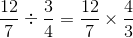In this case, you can reduce the fractions being multiplied by cross-canceling before multiplying them together.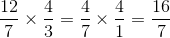### Example Question #6 : How To Divide Fractions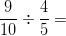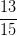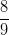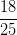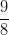Explanation:

Turn the second fraction upside down to find its reciprocal, and then multiply the first fraction by the reciprocal of the second fraction to divide the two fractions. When multiplying fractions, you can multiply across, finding the products of the numbers being multiplied in the numerator and in the denominator.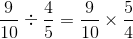In this case, you can reduce the fractions being multiplied by cross-canceling before multiplying them together.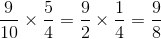### Example Question #1271 : Ssat Upper Level Quantitative (Math)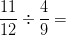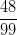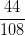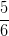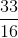Explanation:

Turn the second fraction upside down to find its reciprocal, and then multiply the first fraction by the reciprocal of the second fraction to divide the two fractions. When multiplying fractions, you can multiply across, finding the products of the numbers being multiplied in the numerator and in the denominator.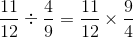In this case, you can reduce the fractions being multiplied by cross-canceling before multiplying them together.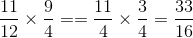### Example Question #1272 : Ssat Upper Level Quantitative (Math)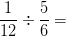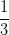Explanation:

Turn the second fraction upside down to find its reciprocal, and then multiply the first fraction by the reciprocal of the second fraction to divide the two fractions. When multiplying fractions, you can multiply across, finding the products of the numbers being multiplied in the numerator and in the denominator.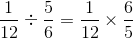In this case, you can reduce the fractions being multiplied by cross-canceling before multiplying them together.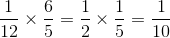### Example Question #1 : How To Divide Fractions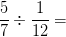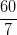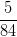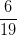Explanation:

Turn the second fraction upside down to find its reciprocal, and then multiply the first fraction by the reciprocal of the second fraction to divide the two fractions. When multiplying fractions, you can multiply across, finding the products of the numbers being multiplied in the numerator and in the denominator.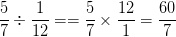### Example Question #1274 : Ssat Upper Level Quantitative (Math)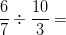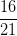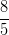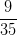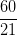Explanation:

Turn the second fraction upside down to find its reciprocal, and then multiply the first fraction by the reciprocal of the second fraction to divide the two fractions. When multiplying fractions, you can multiply across, finding the products of the numbers being multiplied in the numerator and in the denominator.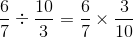In this case, you can reduce the fractions being multiplied by cross-canceling before multiplying them together.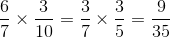← Previous 1